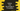# 4 ways to find the average of 10 numbers in Python## How to find the average of 10 numbers in Python:

This post will show you how to find the average of 10 numbers in Python. To get the average value, we need to divide the sum of the numbers by the total count of numbers. So, if we are finding the average of 10 numbers, we need to divide the sum of these numbers by 10.

In this post, we will learn how to find the average of 10 numbers in python in different ways.

### Algorithm to follow:

For example, if n1, n2n10 are 10 given numbers, the average value is calculated by using the below formula:

``(n1 + n2 + n3 + n4 + n5 + n6 + n7 + n8 + n9 + n10)/10``

So, we need to find the sum of the numbers and we can divide the sum by 10 to get the average value.

### Method 1: Find the average by using a for loop:

Let’s use a for loop to get the numbers as input and find the average value:

``````sum = 0

for _ in range(10):
n = float(input('Enter a number: '))
sum = sum + n

average = sum/10

print(f'The average of these numbers is: {average}')``````

In this example,

• The for loop will run for 10 times.
• For each iteration, we are asking the user to enter a number and that number is stored in n.
• n is added to sum. Once the loop ends, sum will hold the total of all of these numbers.
• The average is calculated by dividing sum by 10.
• The last line prints the calculated average value.

If you run this program, it will print output as like below:

``````Enter a number: 10
Enter a number: 12
Enter a number: 122
Enter a number: 12.34
Enter a number: 43.2
Enter a number: 12.333
Enter a number: 77.10
Enter a number: 98
Enter a number: 89.22
Enter a number: 90.1
The average of these numbers is: 56.6293``````

### Method 2: Find average using a while loop:

We can also use a while loop to find the average value. The while loop will run 10 times.

``````sum = 0
count = 0

while count<10:
n = float(input('Enter a number: '))
sum = sum + n
count = count + 1

average = sum/10

print(f'The average of these numbers is: {average}')``````

Here, we have initialized a variable count as 0 and this is used in the while loop. The while loop will run until the value of count is less than 10. Inside the loop, we are incrementing the value of count by 1 on each iteration.

If you run this program, it will give a similar result.

### Method 3: Find the average value without storing the number in a variable:

We can calculate the sum without storing the number value in a separate variable. We need to add the user input value to the sum variable directly without storing it to n first.

Let’s write it using a for loop:

``````sum = 0

for _ in range(10):
sum = sum + float(input('Enter a number: '))

average = sum/10

print(f'The average of these numbers is: {average}')``````

In this program, the user input number is added to sum directly. If you run this program, it will give similar output.

``````Enter a number: 1
Enter a number: 2
Enter a number: 3
Enter a number: 4
Enter a number: 5
Enter a number: 6
Enter a number: 7
Enter a number: 8
Enter a number: 9
Enter a number: 10
The average of these numbers is: 5.5``````

### Method 4: Find the average of numbers in a list:

If the numbers are in a list, we can iterate through the numbers to find the sum and average:

``````sum = 0

given_nums = [1, 2, 3, 4, 5, 6, 7, 8, 9, 10]

for i in given_nums:
sum = sum + i

average = sum/10

print(f'The average of these numbers is: {average}')``````

We can also use the sum method to find the sum of the numbers in a list:

``````given_nums = [1, 2, 3, 4, 5, 6, 7, 8, 9, 10]

average = sum(given_nums)/10

print(f'The average of these numbers is: {average}')``````

It will print the same result.